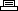Site SearchRainbow SixPrinter Friendly Version

```Level select:
Pause game play, then hold L1 and press Circle, X, Circle, Square,
Square, Triangle, X, X. Note: This may also be done at the main menu.

Invincibility:
Pause game play, then hold L1 and press Square, X, Triangle,
Triangle, X, Square, Circle, Circle. Note: This may also be done at

All main guns:
Pause game play, then hold L1 and press X, Circle(2), Triangle,
Square, X, Square, Circle. Note: This may also be done at the main

All secondary pistols:
Pause game play, then hold L1 and press X, Triangle, Square,
Circle, Square, X, Triangle, Square. Note: This may also be done at

More ammunition:
Pause game play, then hold L1 and press Square(2), Circle, Triangle,
X, Triangle, X, Triangle. Note: This may also be done at the main

Full health for entire team:
Pause game play, then hold L1 and press Triangle(2), X, Circle(2),
X, Square(2). Note: This may also be done at the main menu.

Hostages cannot be killed:
Pause game play, then hold L1 and press Circle(2), Square, Triangle,
X, Triangle, X, Circle. Note: This may also be done at the main menu.

No terrorists:
Pause game play, then hold L1 and press Triangle, Circle(2),
Triangle, Square, X, Triangle, Circle. Note: This may also be done
at the main menu.

Supermen mode:
Pause game play, then hold L1 and press X, Square, Triangle(2),
Circle, Square, X(2). Note: This may also be done at the main menu.

Free-for-all mode:
Pause game play, then hold L1 and press Circle, Triangle(2), X,
Circle, Square, X, Triangle. Note: This may also be done at the main

Open all doors:
Pause game play, then hold L1 and press Triangle, Square(2),
Triangle, X, Circle, Square, Triangle. Note: This may also be done
at the main menu.

Carry all items:
Pause game play, then hold L1 and press Triangle, X, X, Circle,
Square, Circle, X, Triangle. Note: This may also be done at the